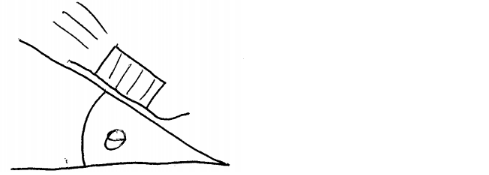# Problem: A box on a sled slides down a long, snow-overed slope. The hill slopes at a constant angle θ. There is a small coefficient of kinetic friction of 0.16. Find an expression for the acceleration.

###### FREE Expert Solution
93% (269 ratings)
###### Problem Details

A box on a sled slides down a long, snow-overed slope. The hill slopes at a constant angle θ. There is a small coefficient of kinetic friction of 0.16. Find an expression for the acceleration.Frequently Asked Questions

What scientific concept do you need to know in order to solve this problem?

Our tutors have indicated that to solve this problem you will need to apply the Inclined Planes with Friction concept. You can view video lessons to learn Inclined Planes with Friction. Or if you need more Inclined Planes with Friction practice, you can also practice Inclined Planes with Friction practice problems.

How long does this problem take to solve?

Our expert Physics tutor, Juan took 3 minutes and 45 seconds to solve this problem. You can follow their steps in the video explanation above.